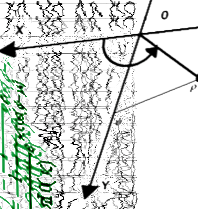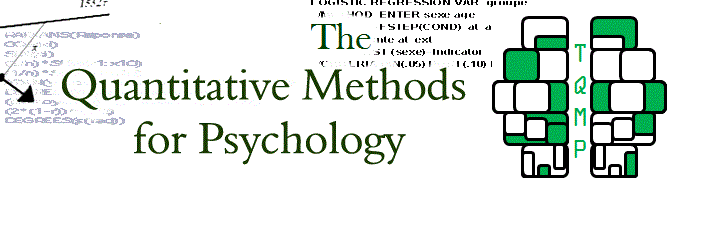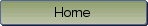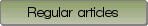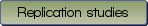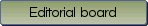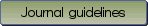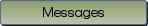Le quotient de deux variances corr\'el\'ees, sa distribution et son test

Bibliographic information: BibTEX format RIS format XML format APA style
Cited references information: BibTEX format APA style

Laurencelle, Louis
131-137
Keywords: Correlated variances , t-test on correlated variances
(no sample data)   (no appendix)

The joint sampling distribution of two correlated variances, i.e. variances stemming from a bivariate normal distribution or from two normal $\rho$-correlated distributions, is hardly known and used, by contrast with the distribution of $F$, the quotient of two independent, zero-correlated variances. The distribution of $F_\rho$, the quotient of two correlated variances, established by Bose (1935) and Finney (1938), is given along with its main characteristics, to which is added a handy $F_\rho$ to $F$ transformation. Finally, data based on Monte Carlo simulations document and compare the accuracy and power of two approximate tests of the difference between two correlated sample variances.

Be informed of the upcoming issues with RSS feed:RSS